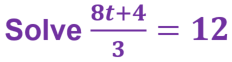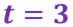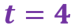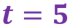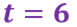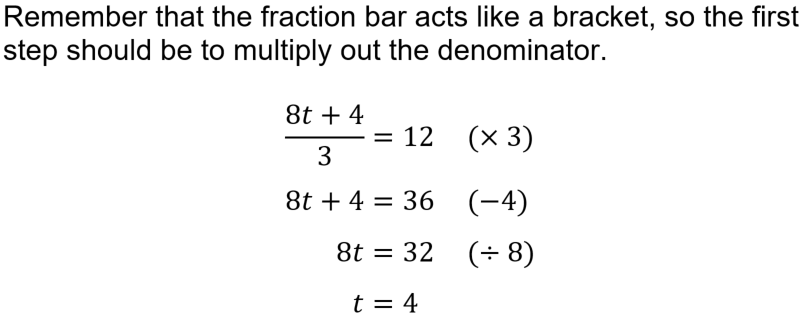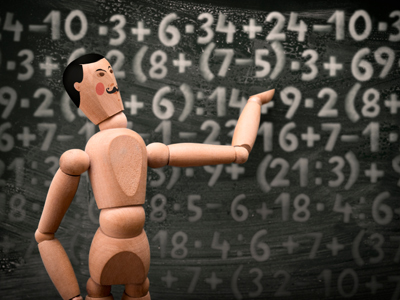Test your skills with linear equations.

# Linear Equations (Numerical) (F)

Solving equations is all about finding out the value of a variable. In a linear equation, so called because it forms a straight line when drawn on a graph, the highest power of the variable (usually x), is just 1. This means that there is only one solution to the equation. How good are you at solving equations? Try this GCSE Maths quiz and find out!

The key to solving equations is to keep it balanced. One way to do this is to imagine an old-fashioned set of weight scales, with the equal sign being the pivot in the middle. If you remove something from one side the scales will tip, unless you also remove it from the other side. You are aiming to reduce everything so that the variable is on one side of the equal sign, and the numbers are on the other.

An alternative is the ‘cover-up’ method. This is best explained using an example, 2x + 3 = 11. Cover up the x-term with your finger, and replace it with ‘what’. In our example this becomes ‘what plus 3 equals 11?’. The next line becomes what was covered up and the answer, so 2x = 8. This easily leads to the final answer, x = 4. This method is a bit harder to apply when the variable is on both sides of the equal sign however.

What is your favourite method? Try them both out, then test yourself in this quiz!

Question 1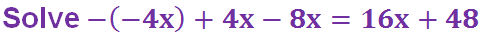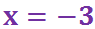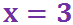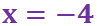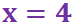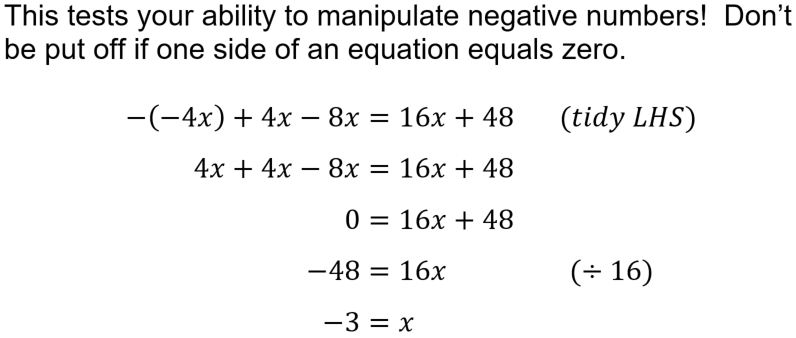Question 2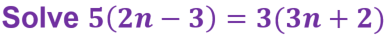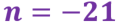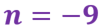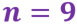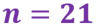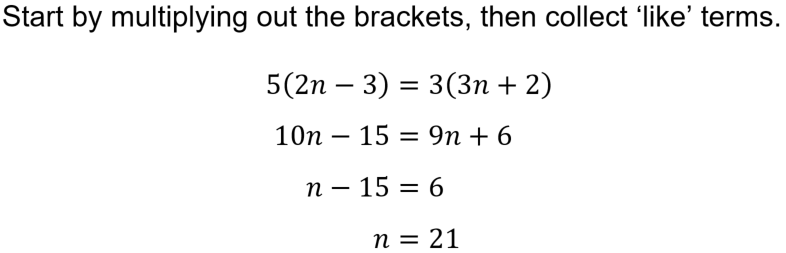Question 3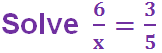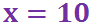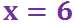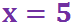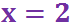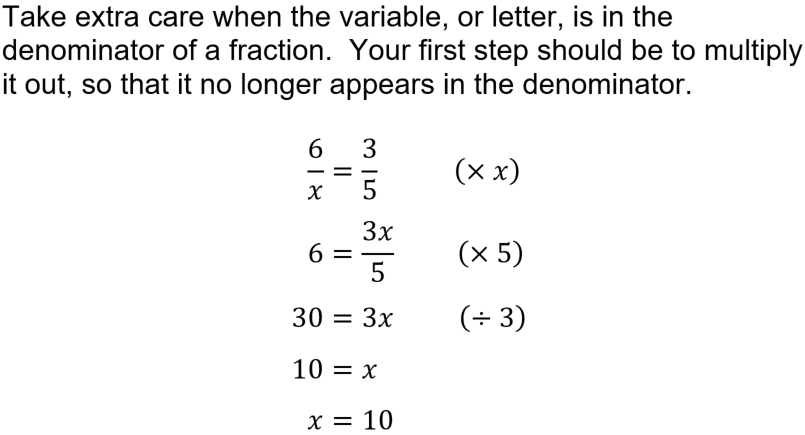Question 4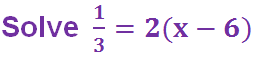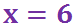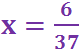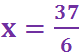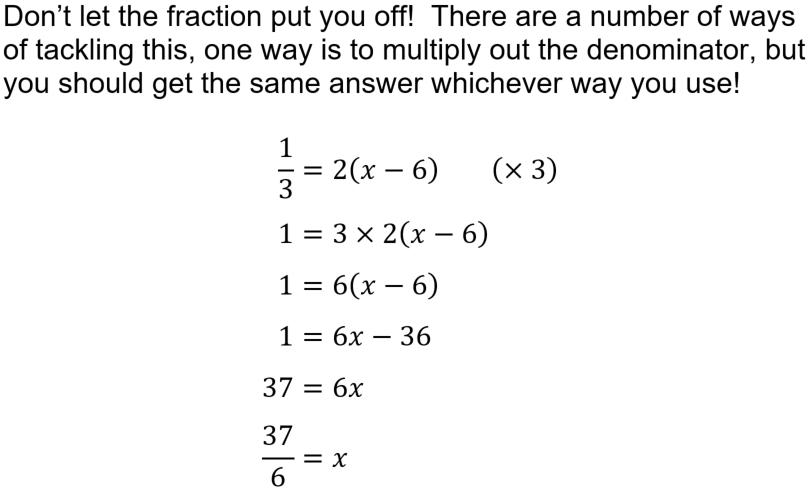Question 5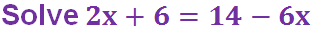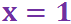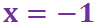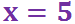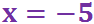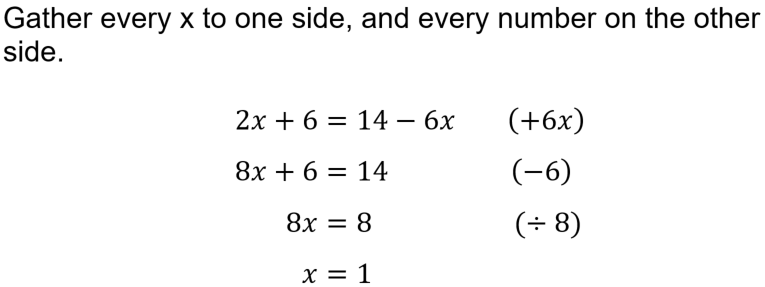Question 6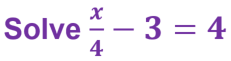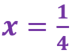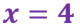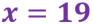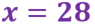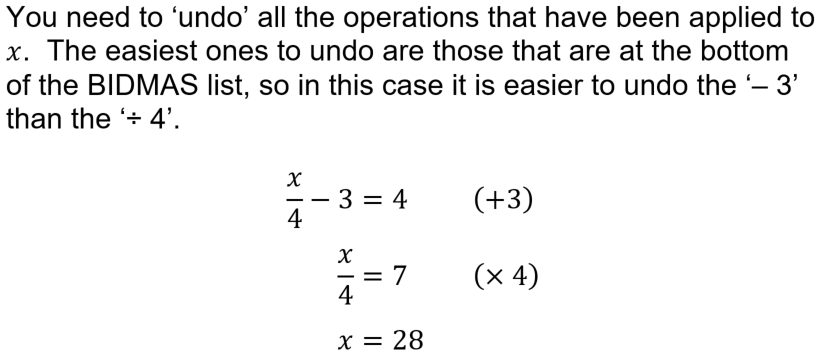Question 7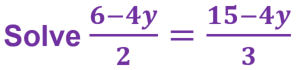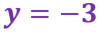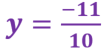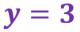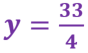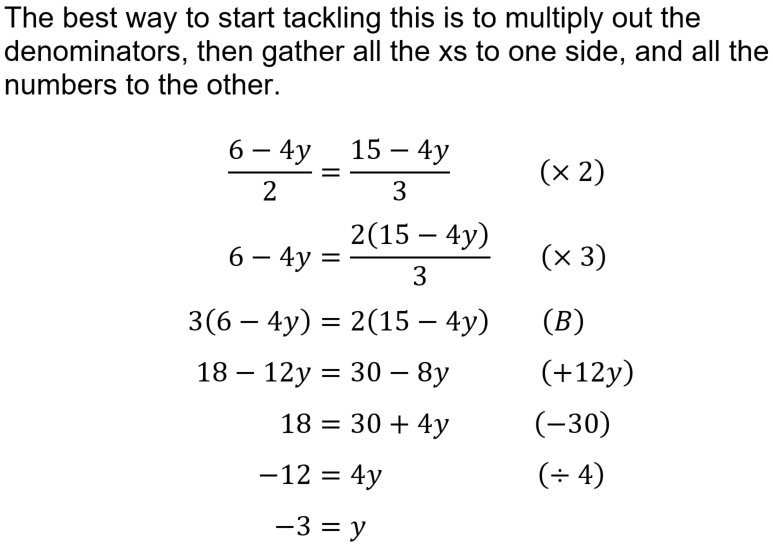Question 8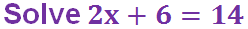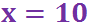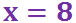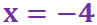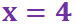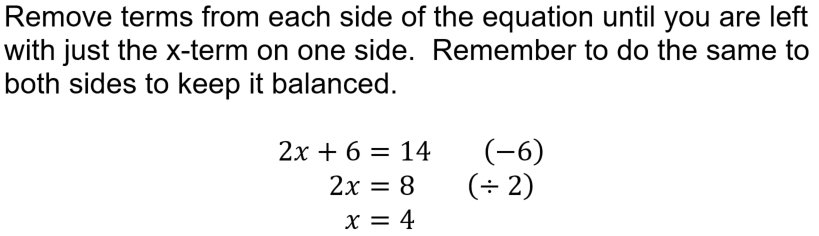Question 9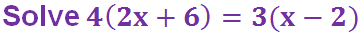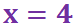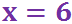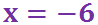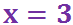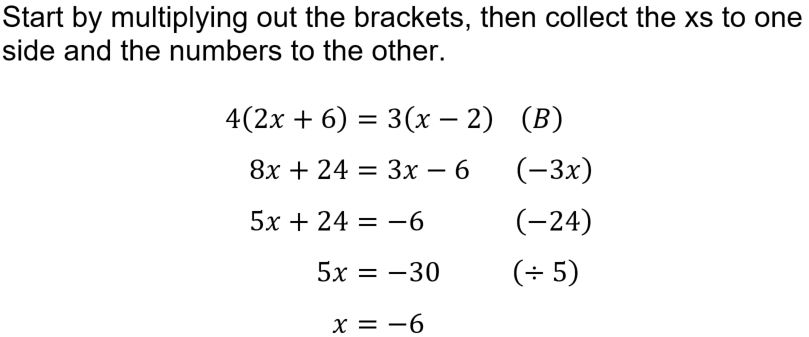Question 10